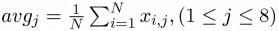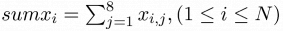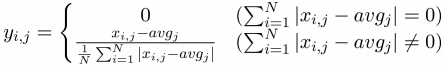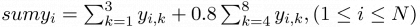## Day 1, Problem 1 - Competition Ranking

A city organized an all-around high school science Olympiad where N competitors (with ID's from 1 to N) each participate in 8 competitions in the subjects of mathematics, physics, chemistry, astronomy, geography, biology, informatics, and linguistics. In the end, scores are taken from all of these fields to produce an overall ranking of the competitors.

Let xi,j denote the rank of competitor i in the j-th competition (1 ≤ iN, 1 ≤ j ≤ 8).

• The average score of the j-th competition is$\textstyle avg_{j}=\frac{1}{N}\sum_{i=1}^{N}x_{i,j}, (1 \leq j \leq 8)$.
• The overall score of competitor i is$\textstyle sumx_{i}=\sum_{j=1}^{8}x_{i,j},(1 \leq i \leq N)$.
• The rank of competitor i in the j-th competition is:$\displaystyle y_{i,j}=\left\{\begin{matrix} 0 & (\sum_{i=1}^{N}|x_{i,j}-avg_{j}|=0) \\ \frac{x_{i,j}-avg_{j}}{\frac{1}{N}\sum_{i=1}^{N}|x_{i,j}-avg_{j}|} & (\sum_{i=1}^{N}|x_{i,j}-avg_{j}| \neq 0) \end{matrix}\right.$
• The overall position score for competitor i is$\textstyle sumy_{i} = \sum_{k=1}^{3}y_{i,k} + 0.8\sum_{k=4}^{8}y_{i,k}, (1 \leq i \leq N)$.

The rules for ranking are as follows:

1. Competitors with higher overall position scores are ranked higher.
2. If two or more competitors have the same overall position score, then the competitor(s) with a higher overall score is ranked higher.
3. If two or more competitors have the same overall position score and the overall score, then the competitor(s) with the smaller ID is ranked higher.

Please write a program for the organizers to compute the rankings of competitors.

### Input Format

The first line of input contains the integer N (1 ≤ N ≤ 1000). The following N lines will each contain 8 space-separated integers xi,1, xi,2, …, xi,8, each between 0 and 100, representing the scores of each competitors in the 8 competitions.

### Output Format

The output should contain N lines, where line i contains the ID of the competitor with rank i (for 1 ≤ iN).

### Sample Input

3
72 82 73 68 95 86 82 90
72 90 50 60 80 70 65 80
72 82 73 68 95 86 82 90


### Sample Output

1
3
2


Point Value: 7
Time Limit: 0.50s
Memory Limit: 16M